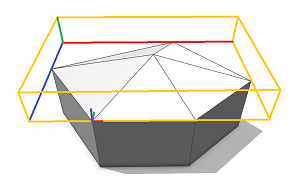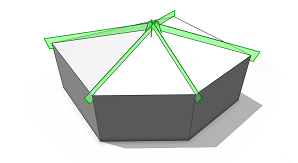# roofPyramid

### Syntax

• roofPyramid(angle)
• roofPyramid(valueType, value)

### Parameters

1. anglefloat
Angle of the roof-planes generation (byAngle).
2. valueTypeselector
{ byAngle | byHeight }—Type of roof generation.
3. valuefloat
Angle or height of the roof-planes as specified by valueType.

### Description

The roofPyramid operation builds a pyramid roof perpendicular to each face of the current shape’s geometry. The polygon center (average of all vertices) gets extruded along the face normal by the given height and connected to all polygon vertices. The new triangles are the roof faces. If an angle is given the height is chosen such that the angle between roof triangle 1 and the polygon is as specified.

##### Note:

The connectivity of the roof mesh is optimized for trim plane generation to cut bricks inserted into the roof planes (see examples below).

### Scope

The scope orientation is set in the following way:

• x-axis direction is kept as much as possible (old x-axis is projected to the plane of the first face).
• y-axis along the face normal of the first face.
• z-axis normal to the two above.

The scope’s sizes are adjusted to tightly fit the extruded geometry.

#### Simple Pyramid Roof

 A pyramid roof with roof slope 30 degrees is built on top of an extruded lot. Note the setting of the pivot and scope. ``````Lot --> extrude(10) Mass Mass --> comp(f) { top : Top | all : X } Top --> roofPyramid(30) Roof``````After a component split, each roof face contains trim planes to cut bricks on insertion. ``````Roof --> comp(f) { all : X }``````##### Note:

There is exactly one roof face per Top shape edge.